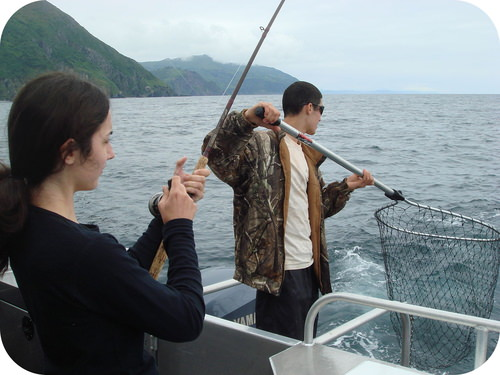# 3.15: Rounding

$$\newcommand{\vecs}{\overset { \rightharpoonup} {\mathbf{#1}} }$$ $$\newcommand{\vecd}{\overset{-\!-\!\rightharpoonup}{\vphantom{a}\smash {#1}}}$$$$\newcommand{\id}{\mathrm{id}}$$ $$\newcommand{\Span}{\mathrm{span}}$$ $$\newcommand{\kernel}{\mathrm{null}\,}$$ $$\newcommand{\range}{\mathrm{range}\,}$$ $$\newcommand{\RealPart}{\mathrm{Re}}$$ $$\newcommand{\ImaginaryPart}{\mathrm{Im}}$$ $$\newcommand{\Argument}{\mathrm{Arg}}$$ $$\newcommand{\norm}{\| #1 \|}$$ $$\newcommand{\inner}{\langle #1, #2 \rangle}$$ $$\newcommand{\Span}{\mathrm{span}}$$ $$\newcommand{\id}{\mathrm{id}}$$ $$\newcommand{\Span}{\mathrm{span}}$$ $$\newcommand{\kernel}{\mathrm{null}\,}$$ $$\newcommand{\range}{\mathrm{range}\,}$$ $$\newcommand{\RealPart}{\mathrm{Re}}$$ $$\newcommand{\ImaginaryPart}{\mathrm{Im}}$$ $$\newcommand{\Argument}{\mathrm{Arg}}$$ $$\newcommand{\norm}{\| #1 \|}$$ $$\newcommand{\inner}{\langle #1, #2 \rangle}$$ $$\newcommand{\Span}{\mathrm{span}}$$$$\newcommand{\AA}{\unicode[.8,0]{x212B}}$$Figure $$\PageIndex{1}$$ (Credit: Nancy Heise; Source: http://commons.wikimedia.org/wiki/File:Catching_salmon_in_the_ocean_off_Raspberry_Island.JPG(opens in new window); License: Public Domain)

### Have you ever been fishing

People who fish often are a little unreliable when it comes to describing what fish they caught and how much it weighed. It's easier to say that the fish weighed ten pounds than it is to accurately describe the weight of 8 pounds 11 ounces. Ten pounds is "close enough" when a fisherman is talking about their catch.

## Rounding

Before dealing with the specifics of the rules for determining the significant figures in a calculated result, we need to be able to round numbers correctly. To round a number, first decide how many significant figures the number should have. Once you know that, round to that many digits, starting from the left. If the number immediately to the right of the last significant digit is less than 5, it is dropped and the value of the last significant digit remains the same. If the number immediately to the right of the last significant digit is greater than or equal to 5, the last significant digit is increased by 1.

Consider the measurement $$207.518 \: \text{m}$$. Right now, the measurement contains six significant figures. How would we successively round it to fewer and fewer significant figures? Follow the process as outlined in Table $$\PageIndex{1}$$.

Table $$\PageIndex{1}$$: Rounding examples
Number of Significant Figures Rounded Value Reasoning
6 207.518 All digits are significant
5 207.52 8 rounds the 1 up to 2
4 207.5 2 is dropped
3 208 5 rounds the 7 up to 8
2 210 8 is replaced by a 0 and rounds the 0 up to 1
1 200 1 is replaced by a 0

Notice that the more rounding that is done, the less reliable the figure is. An approximate value may be sufficient for some purposes, but scientific work requires a much higher level of detail.

## Summary

• Rounding involves the adjustment of a value to account for the proper number of significant digits.
• Rules exist for rounding numbers.

## Review

1. Why do we round numbers?
2. What do we need to know before we round a number?
3. What is “rounding up”?
4. What is “rounding down”?

This page titled 3.15: Rounding is shared under a CK-12 license and was authored, remixed, and/or curated by CK-12 Foundation via source content that was edited to the style and standards of the LibreTexts platform; a detailed edit history is available upon request.